# Diffusion kernels on SNP data embedded in a non-Euclidean metric

Jun. 27, 2014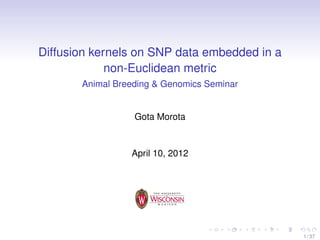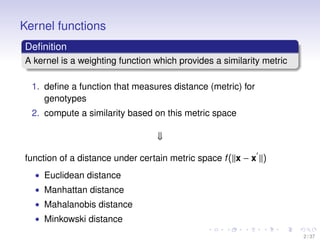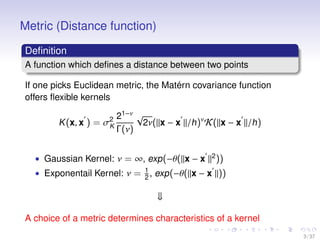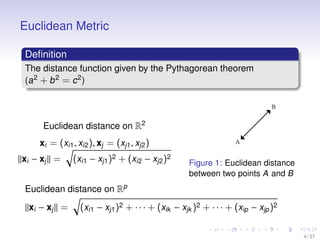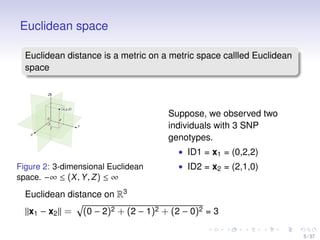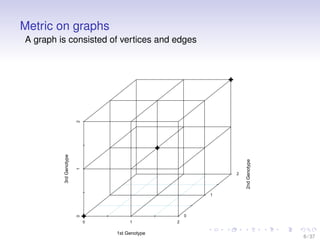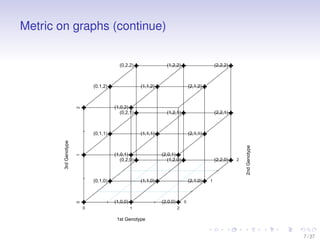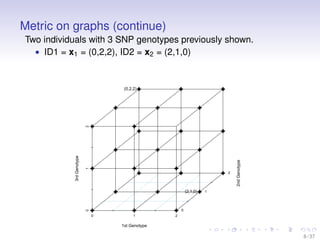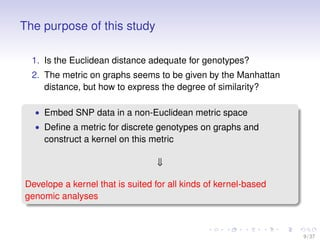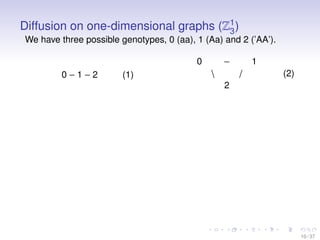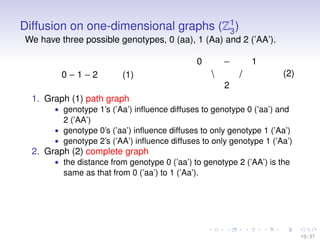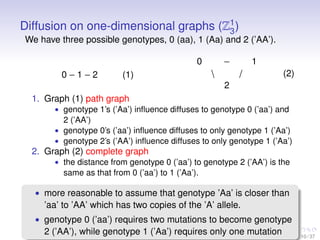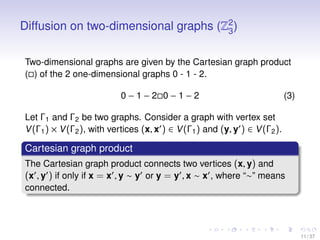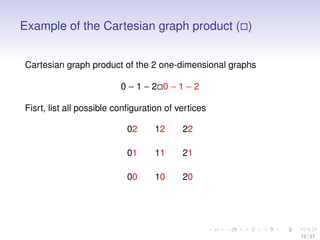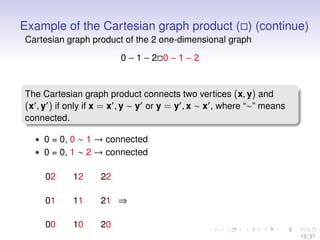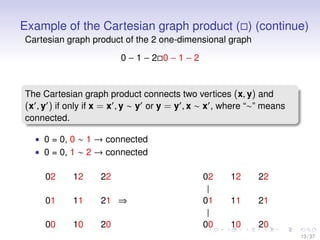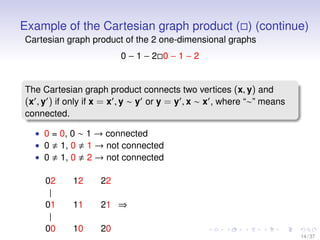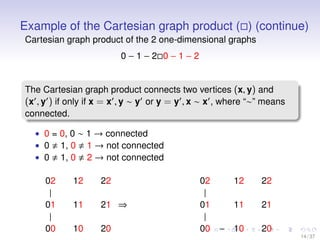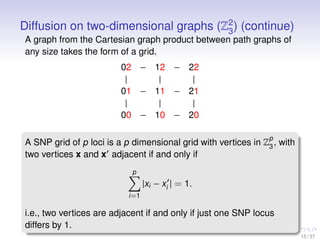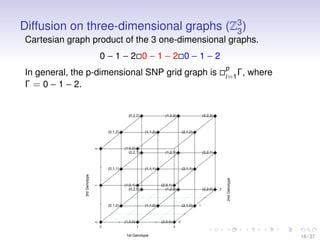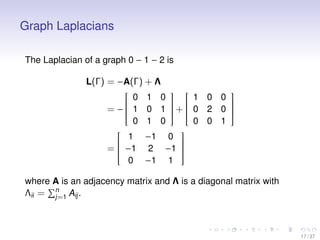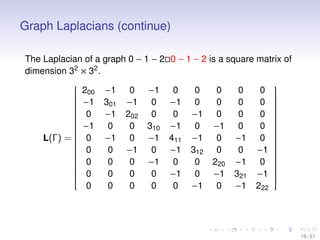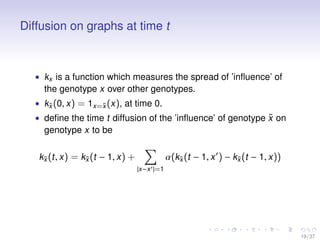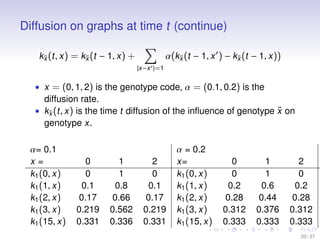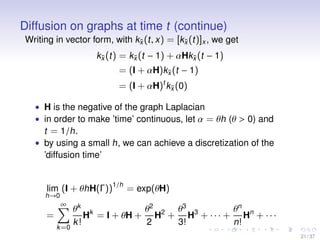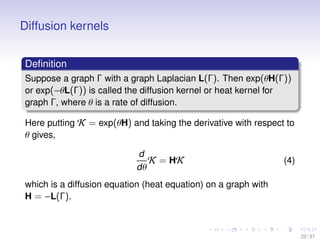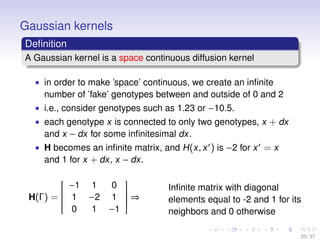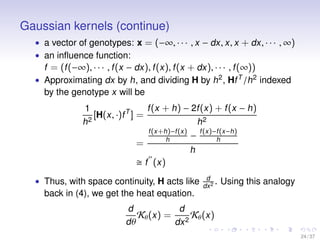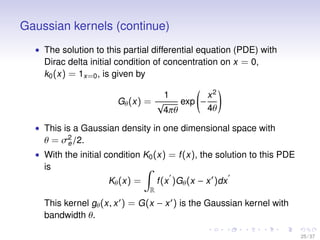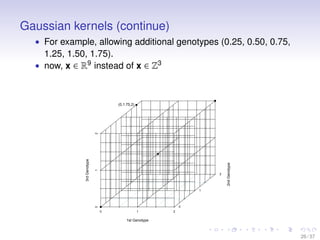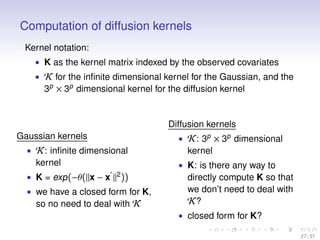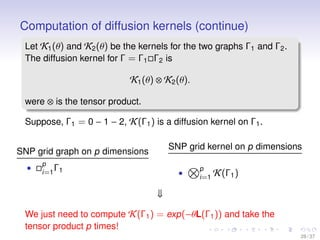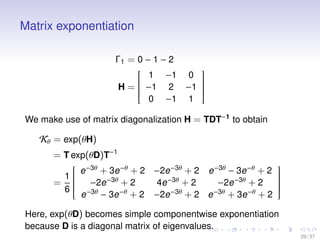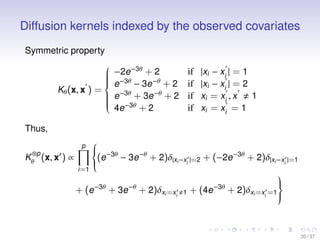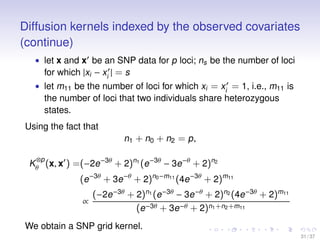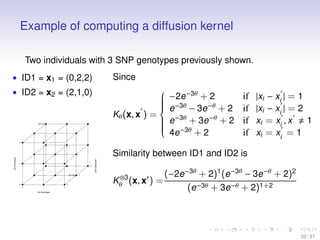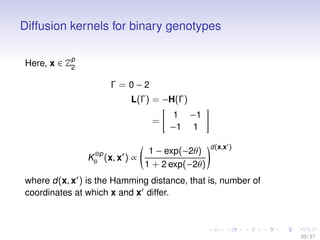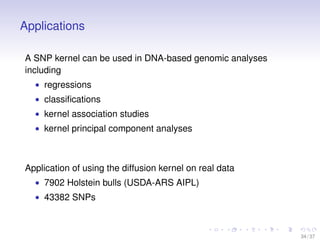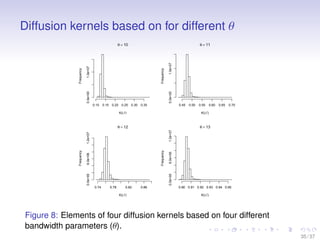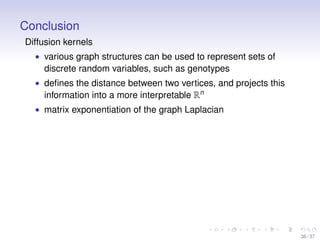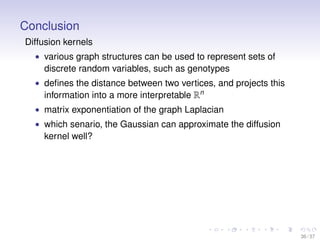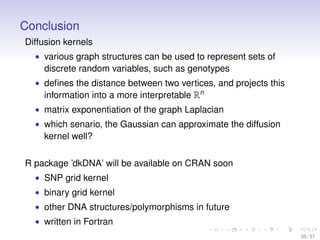1 of 45

### Diffusion kernels on SNP data embedded in a non-Euclidean metric

• 1. Diffusion kernels on SNP data embedded in a non-Euclidean metric Animal Breeding & Genomics Seminar Gota Morota April 10, 2012 1 / 37
• 2. Kernel functions Deﬁnition A kernel is a weighting function which provides a similarity metric 1. deﬁne a function that measures distance (metric) for genotypes 2. compute a similarity based on this metric space ⇓ function of a distance under certain metric space f(||x − x ||) • Euclidean distance • Manhattan distance • Mahalanobis distance • Minkowski distance 2 / 37
• 3. Metric (Distance function) Deﬁnition A function which deﬁnes a distance between two points If one picks Euclidean metric, the Mat´ern covariance function offers ﬂexible kernels K(x, x ) = σ2 K 21−ν Γ(ν) √ 2ν(||x − x ||/h)ν K(||x − x ||/h) • Gaussian Kernel: ν = ∞, exp(−θ(||x − x ||2 )) • Exponentail Kernel: ν = 1 2 , exp(−θ(||x − x ||)) ⇓ A choice of a metric determines characteristics of a kernel 3 / 37
• 4. Euclidean Metric Deﬁnition The distance function given by the Pythagorean theorem (a2 + b2 = c2 ) Euclidean distance on R2 xi = (xi1, xi2), xj = (xj1, xj2) ||xi − xj|| = (xi1 − xj1)2 + (xi2 − xj2)2 Figure 1: Euclidean distance between two points A and B Euclidean distance on Rp ||xi − xj|| = (xi1 − xj1)2 + · · · + (xik − xjk )2 + · · · + (xip − xjp)2 4 / 37
• 5. Euclidean space Euclidean distance is a metric on a metric space callled Euclidean space Figure 2: 3-dimensional Euclidean space. −∞ ≤ (X, Y, Z) ≤ ∞ Suppose, we observed two individuals with 3 SNP genotypes. • ID1 = x1 = (0,2,2) • ID2 = x2 = (2,1,0) Euclidean distance on R3 ||x1 − x2|| = (0 − 2)2 + (2 − 1)2 + (2 − 0)2 = 3 5 / 37
• 6. Metric on graphs A graph is consisted of vertices and edges 0 1 2 012 0 1 2 1st Genotype 2ndGenotype 3rdGenotype 6 / 37
• 7. Metric on graphs (continue) 0 1 2 012 0 1 2 1st Genotype 2ndGenotype 3rdGenotype (2,1,2) (2,0,1) (0,1,2) (0,2,0) (0,1,0) (0,1,1) (1,0,0) (2,0,0) (1,1,0) (2,1,0) (1,2,0) (2,2,0) (1,0,1) (1,1,1) (2,1,1) (0,2,1) (1,2,1) (2,2,1) (0,2,2) (1,2,2) (2,2,2) (1,0,2) (1,1,2) 7 / 37
• 8. Metric on graphs (continue) Two individuals with 3 SNP genotypes previously shown. • ID1 = x1 = (0,2,2), ID2 = x2 = (2,1,0) 0 1 2 012 0 1 2 1st Genotype 2ndGenotype 3rdGenotype (2,1,0) (0,2,2) 8 / 37
• 9. The purpose of this study 1. Is the Euclidean distance adequate for genotypes? 2. The metric on graphs seems to be given by the Manhattan distance, but how to express the degree of similarity? • Embed SNP data in a non-Euclidean metric space • Deﬁne a metric for discrete genotypes on graphs and construct a kernel on this metric ⇓ Develope a kernel that is suited for all kinds of kernel-based genomic analyses 9 / 37
• 10. Diffusion on one-dimensional graphs (Z1 3) We have three possible genotypes, 0 (aa), 1 (Aa) and 2 (’AA’). 0 − 1 − 2 (1) 0 − 1 / 2 (2) 10 / 37
• 11. Diffusion on one-dimensional graphs (Z1 3) We have three possible genotypes, 0 (aa), 1 (Aa) and 2 (’AA’). 0 − 1 − 2 (1) 0 − 1 / 2 (2) 1. Graph (1) path graph • genotype 1’s (’Aa’) inﬂuence diffuses to genotype 0 (’aa’) and 2 (’AA’) • genotype 0’s (’aa’) inﬂuence diffuses to only genotype 1 (’Aa’) • genotype 2’s (’AA’) inﬂuence diffuses to only genotype 1 (’Aa’) 2. Graph (2) complete graph • the distance from genotype 0 (’aa’) to genotype 2 (’AA’) is the same as that from 0 (’aa’) to 1 (’Aa’). 10 / 37
• 12. Diffusion on one-dimensional graphs (Z1 3) We have three possible genotypes, 0 (aa), 1 (Aa) and 2 (’AA’). 0 − 1 − 2 (1) 0 − 1 / 2 (2) 1. Graph (1) path graph • genotype 1’s (’Aa’) inﬂuence diffuses to genotype 0 (’aa’) and 2 (’AA’) • genotype 0’s (’aa’) inﬂuence diffuses to only genotype 1 (’Aa’) • genotype 2’s (’AA’) inﬂuence diffuses to only genotype 1 (’Aa’) 2. Graph (2) complete graph • the distance from genotype 0 (’aa’) to genotype 2 (’AA’) is the same as that from 0 (’aa’) to 1 (’Aa’). • more reasonable to assume that genotype ’Aa’ is closer than ’aa’ to ’AA’ which has two copies of the ’A’ allele. • genotype 0 (’aa’) requires two mutations to become genotype 2 (’AA’), while genotype 1 (’Aa’) requires only one mutation 10 / 37
• 13. Diffusion on two-dimensional graphs (Z2 3) Two-dimensional graphs are given by the Cartesian graph product ( ) of the 2 one-dimensional graphs 0 - 1 - 2. 0 − 1 − 2 0 − 1 − 2 (3) Let Γ1 and Γ2 be two graphs. Consider a graph with vertex set V(Γ1) × V(Γ2), with vertices (x, x ) ∈ V(Γ1) and (y, y ) ∈ V(Γ2). Cartesian graph product The Cartesian graph product connects two vertices (x, y) and (x , y ) if only if x = x , y ∼ y or y = y , x ∼ x , where “∼” means connected. 11 / 37
• 14. Example of the Cartesian graph product ( ) Cartesian graph product of the 2 one-dimensional graphs 0 − 1 − 2 0 − 1 − 2 Fisrt, list all possible conﬁguration of vertices 02 12 22 01 11 21 00 10 20 12 / 37
• 15. Example of the Cartesian graph product ( ) (continue) Cartesian graph product of the 2 one-dimensional graph 0 − 1 − 2 0 − 1 − 2 The Cartesian graph product connects two vertices (x, y) and (x , y ) if only if x = x , y ∼ y or y = y , x ∼ x , where “∼” means connected. • 0 = 0, 0 ∼ 1 → connected • 0 = 0, 1 ∼ 2 → connected 02 12 22 01 11 21 00 10 20 ⇒ 13 / 37
• 16. Example of the Cartesian graph product ( ) (continue) Cartesian graph product of the 2 one-dimensional graph 0 − 1 − 2 0 − 1 − 2 The Cartesian graph product connects two vertices (x, y) and (x , y ) if only if x = x , y ∼ y or y = y , x ∼ x , where “∼” means connected. • 0 = 0, 0 ∼ 1 → connected • 0 = 0, 1 ∼ 2 → connected 02 12 22 01 11 21 00 10 20 ⇒ 13 / 37
• 17. Example of the Cartesian graph product ( ) (continue) Cartesian graph product of the 2 one-dimensional graph 0 − 1 − 2 0 − 1 − 2 The Cartesian graph product connects two vertices (x, y) and (x , y ) if only if x = x , y ∼ y or y = y , x ∼ x , where “∼” means connected. • 0 = 0, 0 ∼ 1 → connected • 0 = 0, 1 ∼ 2 → connected 02 12 22 01 11 21 00 10 20 ⇒ 02 12 22 | 01 11 21 | 00 10 20 13 / 37
• 18. Example of the Cartesian graph product ( ) (continue) Cartesian graph product of the 2 one-dimensional graphs 0 − 1 − 2 0 − 1 − 2 The Cartesian graph product connects two vertices (x, y) and (x , y ) if only if x = x , y ∼ y or y = y , x ∼ x , where “∼” means connected. • 0 = 0, 0 ∼ 1 → connected • 0 1, 0 1 → not connected • 0 1, 0 2 → not connected 02 12 22 | 01 11 21 | 00 10 20 ⇒ 14 / 37
• 19. Example of the Cartesian graph product ( ) (continue) Cartesian graph product of the 2 one-dimensional graphs 0 − 1 − 2 0 − 1 − 2 The Cartesian graph product connects two vertices (x, y) and (x , y ) if only if x = x , y ∼ y or y = y , x ∼ x , where “∼” means connected. • 0 = 0, 0 ∼ 1 → connected • 0 1, 0 1 → not connected • 0 1, 0 2 → not connected 02 12 22 | 01 11 21 | 00 10 20 ⇒ 14 / 37
• 20. Example of the Cartesian graph product ( ) (continue) Cartesian graph product of the 2 one-dimensional graphs 0 − 1 − 2 0 − 1 − 2 The Cartesian graph product connects two vertices (x, y) and (x , y ) if only if x = x , y ∼ y or y = y , x ∼ x , where “∼” means connected. • 0 = 0, 0 ∼ 1 → connected • 0 1, 0 1 → not connected • 0 1, 0 2 → not connected 02 12 22 | 01 11 21 | 00 10 20 ⇒ 02 12 22 | 01 11 21 | 00 − 10 20 14 / 37
• 21. Diffusion on two-dimensional graphs (Z2 3) (continue) A graph from the Cartesian graph product between path graphs of any size takes the form of a grid. 02 − 12 − 22 | | | 01 − 11 − 21 | | | 00 − 10 − 20 A SNP grid of p loci is a p dimensional grid with vertices in Zp 3 , with two vertices x and x adjacent if and only if p i=1 |xi − xi | = 1. i.e., two vertices are adjacent if and only if just one SNP locus differs by 1. 15 / 37
• 22. Diffusion on three-dimensional graphs (Z3 3 ) Cartesian graph product of the 3 one-dimensional graphs. 0 − 1 − 2 0 − 1 − 2 0 − 1 − 2 In general, the p-dimensional SNP grid graph is p i=1 Γ, where Γ = 0 − 1 − 2. 0 1 2 012 0 1 2 1st Genotype 2ndGenotype 3rdGenotype (2,1,2) (2,0,1) (0,1,2) (0,2,0) (0,1,0) (0,1,1) (1,0,0) (2,0,0) (1,1,0) (2,1,0) (1,2,0) (2,2,0) (1,0,1) (1,1,1) (2,1,1) (0,2,1) (1,2,1) (2,2,1) (0,2,2) (1,2,2) (2,2,2) (1,0,2) (1,1,2) 16 / 37
• 23. Graph Laplacians The Laplacian of a graph 0 − 1 − 2 is L(Γ) = −A(Γ) + Λ = −   0 1 0 1 0 1 0 1 0   +   1 0 0 0 2 0 0 0 1   =   1 −1 0 −1 2 −1 0 −1 1   where A is an adjacency matrix and Λ is a diagonal matrix with Λii = n j=1 Aij. 17 / 37
• 24. Graph Laplacians (continue) The Laplacian of a graph 0 − 1 − 2 0 − 1 − 2 is a square matrix of dimension 32 × 32 . L(Γ) =   200 −1 0 −1 0 0 0 0 0 −1 301 −1 0 −1 0 0 0 0 0 −1 202 0 0 −1 0 0 0 −1 0 0 310 −1 0 −1 0 0 0 −1 0 −1 411 −1 0 −1 0 0 0 −1 0 −1 312 0 0 −1 0 0 0 −1 0 0 220 −1 0 0 0 0 0 −1 0 −1 321 −1 0 0 0 0 0 −1 0 −1 222   18 / 37
• 25. Diffusion on graphs at time t • kx is a function which measures the spread of ’inﬂuence’ of the genotype x over other genotypes. • k˜x(0, x) = 1x=˜x(x), at time 0. • deﬁne the time t diffusion of the ’inﬂuence’ of genotype ˜x on genotype x to be k˜x(t, x) = k˜x(t − 1, x) + |x−x |=1 α(k˜x(t − 1, x ) − k˜x(t − 1, x)) 19 / 37
• 26. Diffusion on graphs at time t (continue) k˜x(t, x) = k˜x(t − 1, x) + |x−x |=1 α(k˜x(t − 1, x ) − k˜x(t − 1, x)) • x = (0, 1, 2) is the genotype code, α = (0.1, 0.2) is the diffusion rate. • k˜x(t, x) is the time t diffusion of the inﬂuence of genotype ˜x on genotype x. α= 0.1 α = 0.2 x = 0 1 2 x= 0 1 2 k1(0, x) 0 1 0 k1(0, x) 0 1 0 k1(1, x) 0.1 0.8 0.1 k1(1, x) 0.2 0.6 0.2 k1(2, x) 0.17 0.66 0.17 k1(2, x) 0.28 0.44 0.28 k1(3, x) 0.219 0.562 0.219 k1(3, x) 0.312 0.376 0.312 k1(15, x) 0.331 0.336 0.331 k1(15, x) 0.333 0.333 0.333 20 / 37
• 27. Diffusion on graphs at time t (continue) Writing in vector form, with k˜x(t, x) = [k˜x(t)]x, we get k˜x(t) = k˜x(t − 1) + αHk˜x(t − 1) = (I + αH)k˜x(t − 1) = (I + αH)t k˜x(0) • H is the negative of the graph Laplacian • in order to make ’time’ continuous, let α = θh (θ > 0) and t = 1/h. • by using a small h, we can achieve a discretization of the ’diffusion time’ lim h→0 (I + θhH(Γ))1/h = exp(θH) = ∞ k=0 θk k! Hk = I + θH + θ2 2 H2 + θ3 3! H3 + · · · + θn n! Hn + · · · 21 / 37
• 28. Diffusion kernels Deﬁnition Suppose a graph Γ with a graph Laplacian L(Γ). Then exp(θH(Γ)) or exp(−θL(Γ)) is called the diffusion kernel or heat kernel for graph Γ, where θ is a rate of diffusion. Here putting K = exp(θH) and taking the derivative with respect to θ gives, d dθ K = HK (4) which is a diffusion equation (heat equation) on a graph with H = −L(Γ). 22 / 37
• 29. Gaussian kernels Deﬁnition A Gaussian kernel is a space continuous diffusion kernel • in order to make ’space’ continuous, we create an inﬁnite number of ’fake’ genotypes between and outside of 0 and 2 • i.e., consider genotypes such as 1.23 or −10.5. • each genotype x is connected to only two genotypes, x + dx and x − dx for some inﬁnitesimal dx. • H becomes an inﬁnite matrix, and H(x, x ) is −2 for x = x and 1 for x + dx, x − dx. H(Γ) =   −1 1 0 1 −2 1 0 1 −1   ⇒ Inﬁnite matrix with diagonal elements equal to -2 and 1 for its neighbors and 0 otherwise 23 / 37
• 30. Gaussian kernels (continue) • a vector of genotypes: x = (−∞, · · · , x − dx, x, x + dx, · · · , ∞) • an inﬂuence function: f = (f(−∞), · · · , f(x − dx), f(x), f(x + dx), · · · , f(∞)) • Approximating dx by h, and dividing H by h2 , HfT /h2 indexed by the genotype x will be 1 h2 [H(x, ·)fT ] = f(x + h) − 2f(x) + f(x − h) h2 = f(x+h)−f(x) h − f(x)−f(x−h) h h f (x) • Thus, with space continuity, H acts like d dx2 . Using this analogy back in (4), we get the heat equation. d dθ Kθ(x) = d dx2 Kθ(x) 24 / 37
• 31. Gaussian kernels (continue) • The solution to this partial differential equation (PDE) with Dirac delta initial condition of concentration on x = 0, k0(x) = 1x=0, is given by Gθ(x) = 1 √ 4πθ exp − x2 4θ • This is a Gaussian density in one dimensional space with θ = σ2 e/2. • With the initial condition K0(x) = f(x), the solution to this PDE is Kθ(x) = R f(x )Gθ(x − x )dx This kernel gθ(x, x ) = G(x − x ) is the Gaussian kernel with bandwidth θ. 25 / 37
• 32. Gaussian kernels (continue) • For example, allowing additional genotypes (0.25, 0.50, 0.75, 1.25, 1.50, 1.75). • now, x ∈ R9 instead of x ∈ Z3 0 1 2 012 0 1 2 1st Genotype 2ndGenotype 3rdGenotype (0,1.75,2) 26 / 37
• 33. Computation of diffusion kernels Kernel notation: • K as the kernel matrix indexed by the observed covariates • K for the inﬁnite dimensional kernel for the Gaussian, and the 3p × 3p dimensional kernel for the diffusion kernel Gaussian kernels • K: inﬁnite dimensional kernel • K = exp(−θ(||x − x ||2 )) • we have a closed form for K, so no need to deal with K Diffusion kernels • K: 3p × 3p dimensional kernel • K: is there any way to directly compute K so that we don’t need to deal with K? • closed form for K? 27 / 37
• 34. Computation of diffusion kernels (continue) Let K1(θ) and K2(θ) be the kernels for the two graphs Γ1 and Γ2. The diffusion kernel for Γ = Γ1 Γ2 is K1(θ) ⊗ K2(θ). were ⊗ is the tensor product. Suppose, Γ1 = 0 − 1 − 2, K(Γ1) is a diffusion kernel on Γ1. SNP grid graph on p dimensions • p i=1 Γ1 SNP grid kernel on p dimensions • p i=1 K(Γ1) ⇓ We just need to compute K(Γ1) = exp(−θL(Γ1)) and take the tensor product p times! 28 / 37
• 35. Matrix exponentiation Γ1 = 0 − 1 − 2 H =   1 −1 0 −1 2 −1 0 −1 1   We make use of matrix diagonalization H = TDT−1 to obtain Kθ = exp(θH) = T exp(θD)T−1 = 1 6   e−3θ + 3e−θ + 2 −2e−3θ + 2 e−3θ − 3e−θ + 2 −2e−3θ + 2 4e−3θ + 2 −2e−3θ + 2 e−3θ − 3e−θ + 2 −2e−3θ + 2 e−3θ + 3e−θ + 2   Here, exp(θD) becomes simple componentwise exponentiation because D is a diagonal matrix of eigenvalues. 29 / 37
• 36. Diffusion kernels indexed by the observed covariates Symmetric property Kθ(x, x ) =    −2e−3θ + 2 if |xi − xi | = 1 e−3θ − 3e−θ + 2 if |xi − xi | = 2 e−3θ + 3e−θ + 2 if xi = xi , x 1 4e−3θ + 2 if xi = xi = 1 Thus, K ⊗p θ (x, x ) ∝ p i=1    (e−3θ − 3e−θ + 2)δ|xi−xi |=2 + (−2e−3θ + 2)δ|xi−xi |=1 + (e−3θ + 3e−θ + 2)δxi=xi 1 + (4e−3θ + 2)δxi=xi =1    30 / 37
• 37. Diffusion kernels indexed by the observed covariates (continue) • let x and x be an SNP data for p loci; ns be the number of loci for which |xi − xi | = s • let m11 be the number of loci for which xi = xi = 1, i.e., m11 is the number of loci that two individuals share heterozygous states. Using the fact that n1 + n0 + n2 = p, K ⊗p θ (x, x ) =(−2e−3θ + 2)n1 (e−3θ − 3e−θ + 2)n2 (e−3θ + 3e−θ + 2)n0−m11 (4e−3θ + 2)m11 ∝ (−2e−3θ + 2)n1 (e−3θ − 3e−θ + 2)n2 (4e−3θ + 2)m11 (e−3θ + 3e−θ + 2)n1+n2+m11 We obtain a SNP grid kernel. 31 / 37
• 38. Example of computing a diffusion kernel Two individuals with 3 SNP genotypes previously shown. • ID1 = x1 = (0,2,2) • ID2 = x2 = (2,1,0) 0 1 2 012 0 1 2 1st Genotype 2ndGenotype 3rdGenotype (2,1,0) (0,2,2) Since Kθ(x, x ) =    −2e−3θ + 2 if |xi − xi | = 1 e−3θ − 3e−θ + 2 if |xi − xi | = 2 e−3θ + 3e−θ + 2 if xi = xi , x 1 4e−3θ + 2 if xi = xi = 1 Similarity between ID1 and ID2 is K⊗3 θ (x, x ) = (−2e−3θ + 2)1 (e−3θ − 3e−θ + 2)2 (e−3θ + 3e−θ + 2)1+2 32 / 37
• 39. Diffusion kernels for binary genotypes Here, x ∈ Zp 2 Γ = 0 − 2 L(Γ) = −H(Γ) = 1 −1 −1 1 K ⊗p θ (x, x ) ∝ 1 − exp(−2θ) 1 + 2 exp(−2θ) d(x,x ) where d(x, x ) is the Hamming distance, that is, number of coordinates at which x and x differ. 33 / 37
• 40. Applications A SNP kernel can be used in DNA-based genomic analyses including • regressions • classiﬁcations • kernel association studies • kernel principal component analyses Application of using the diffusion kernel on real data • 7902 Holstein bulls (USDA-ARS AIPL) • 43382 SNPs 34 / 37
• 41. Diffusion kernels based on for different θ K(i,i') Frequency 0.10 0.15 0.20 0.25 0.30 0.35 0.0e+001.0e+07 θ = 10 K(i,i') Frequency 0.45 0.50 0.55 0.60 0.65 0.70 0.0e+001.0e+07 θ = 11 K(i,i') Frequency 0.74 0.78 0.82 0.86 0.0e+006.0e+061.2e+07 θ = 12 K(i,i') Frequency 0.90 0.91 0.92 0.93 0.94 0.95 0.0e+006.0e+061.2e+07 θ = 13 Figure 8: Elements of four diffusion kernels based on four different bandwidth parameters (θ). 35 / 37
• 42. Conclusion Diffusion kernels • various graph structures can be used to represent sets of discrete random variables, such as genotypes • deﬁnes the distance between two vertices, and projects this information into a more interpretable Rn • matrix exponentiation of the graph Laplacian 36 / 37
• 43. Conclusion Diffusion kernels • various graph structures can be used to represent sets of discrete random variables, such as genotypes • deﬁnes the distance between two vertices, and projects this information into a more interpretable Rn • matrix exponentiation of the graph Laplacian • which senario, the Gaussian can approximate the diffusion kernel well? 36 / 37
• 44. Conclusion Diffusion kernels • various graph structures can be used to represent sets of discrete random variables, such as genotypes • deﬁnes the distance between two vertices, and projects this information into a more interpretable Rn • matrix exponentiation of the graph Laplacian • which senario, the Gaussian can approximate the diffusion kernel well? R package ’dkDNA’ will be available on CRAN soon • SNP grid kernel • binary grid kernel • other DNA structures/polymorphisms in future • written in Fortran 36 / 37
• 45. Acknowledgments • Daniel Gianola • Grace Wahba • Masanori Koyama • Chen Yao 37 / 37